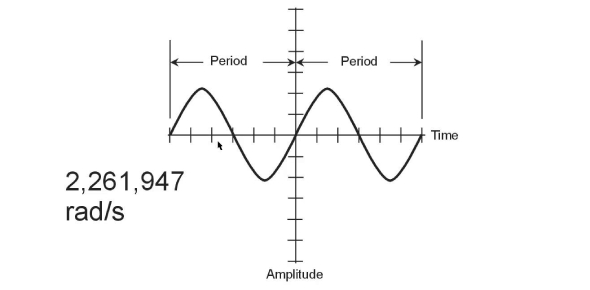# Stan Gibilisco Electronics Book MCQ: Basic DC Circuits

20 Questions | Attempts: 1701SettingsThis is the MCQs for Gibilisco: CHAPTER 4 - BASIC DC CIRCUITS

• 1.
Suppose you double the voltage in a simple dc circuit, and cut the resistance in half. The current will become:
• A.

Four times as great

• B.

Twice as great

• C.

The same as it was before

• D.

Half as great

• 2.
A wiring diagram would most likely be found in:
• A.

An engineer’s general circuit idea notebook

• B.

• C.

• D.

A procedural flowchart

• 3.
Given a dc voltage source delivering 24 V and a circuit resistance of 3.3 KΩ, what is the current?
• A.

0.73 A

• B.

138 A

• C.

138 mA

• D.

7.3 mA

• 4.
Suppose that a circuit has 472 Ω of resistance and the current is 875 mA. Then the source voltage is:
• A.

413 V

• B.

0.539 V

• C.

1.85 V

• D.

None of the above

• 5.
The dc voltage in a circuit is 550 mV and the current is 7.2 mA. Then the resistance is:
• A.

0.76 Ω

• B.

76 Ω

• C.

0.0040 Ω

• D.

None of the above

• 6.
Given a dc voltage source of 3.5 kV and a circuit resistance of 220 Ω, what is the current?
• A.

16 mA

• B.

6.3 mA

• C.

6.3 A

• D.

None of the above

• 7.
A source delivers 12 V and the current is 777 mA. Then the best expression for the resistance is:
• A.

15 Ω

• B.

15.4 Ω

• C.

9.3 Ω

• D.

9.32 Ω

• 8.
A circuit has a total resistance of 473,332 Ω and draws 4.4 mA. The best expression for the voltage of the source is:
• A.

2082 V

• B.

110 kV

• C.

2.1 kV

• D.

2.08266 kV

• 9.
The voltage is 250 V and the current is 8.0 mA. The power dissipated by the potentiometer is:
• A.

31 mW

• B.

31 W

• C.

2.0 W

• D.

2.0 mW

• 10.
The voltage from the source is 12 V and the potentiometer is set for 470 Ω. The power is about:
• A.

310 mW

• B.

25.5 mW

• C.

39.2 W

• D.

3.26 W

• 11.
The current through the potentiometer is 17 mA and its value is 1.22KΩ. The power is:
• A.

0.24 μW

• B.

20.7 W

• C.

20.7 mW

• D.

350 mW

• 12.
Suppose six resistors are hooked up in series, and each of them has a value of 540 Ω. Then the total resistance is:
• A.

90 Ω

• B.

3.24 KΩ

• C.

540 Ω

• D.

None of the above

• 13.
Four resistors are connected in series, each with a value of 4.0 KΩ. The total resistance is:
• A.

1 KΩ

• B.

4 KΩ

• C.

8 KΩ

• D.

16 KΩ

• 14.
Suppose you have three resistors in parallel, each with a value of 68,000 Ω. Then the total resistance is:
• A.

23 Ω

• B.

23 KΩ

• C.

204 Ω

• D.

0.2 MΩ

• 15.
There are three resistors in parallel, with values of 22 Ω, 27Ω, and 33 Ω. A 12-V battery is connected across this combination, as shown in Fig. 4-11. What is the current drawn from the battery by this resistance combination?
• A.

1.3 A

• B.

15 mA

• C.

150 mA

• D.

1.5 A

• 16.
Three resistors, with values of 47 Ω, 68 Ω, and 82 Ω, are connected in series with a 50-V dc generator, as shown in Fig. 4-12. The total power consumed by this network of resistors is:
• A.

250 mW

• B.

13 mW

• C.

13 W

• D.

Not determinable from the data given

• 17.
You have an unlimited supply of 1-W, 100-Ω resistors. You need to get a 100- Ω, 10-W resistor. This can be done most cheaply by means of a series-parallel matrix of
• A.

3 X 3 resistors

• B.

4 X 3 resistors

• C.

4 X 4 resistors

• D.

2 X 5 resistors

• 18.
You have an unlimited supply of 1-W, 1000-Ω resistors, and you need a 500-Ω resistance rated at 7 W or more. This can be done by assembling
• A.

Four sets of two 1000-Ω resistors in series, and connecting these four sets in parallel

• B.

Four sets of two 1000-Ω resistors in parallel, and connecting these four sets in series

• C.

A 3 X 3 series-parallel matrix of 1000-Ω resistors

• D.

Something other than any of the above

• 19.
You have an unlimited supply of 1-W, 1000-Ω resistors, and you need to get a 3000-Ω, 5-W resistance. The best way is to:
• A.

Make a 2 X 2 series-parallel matrix

• B.

Connect three of the resistors in parallel

• C.

Make a 3 X 3 series-parallel matrix

• D.

Do something other than any of the above

• 20.
Good engineering practice usually requires that a series-parallel resistive network be made:
• A.

From resistors that are all very rugged

• B.

From resistors that are all the same

• C.

From a series combination of resistors in parallel

• D.

From a parallel combination of resistors in series

## Related TopicsBack to top
×

Wait!
Here's an interesting quiz for you.